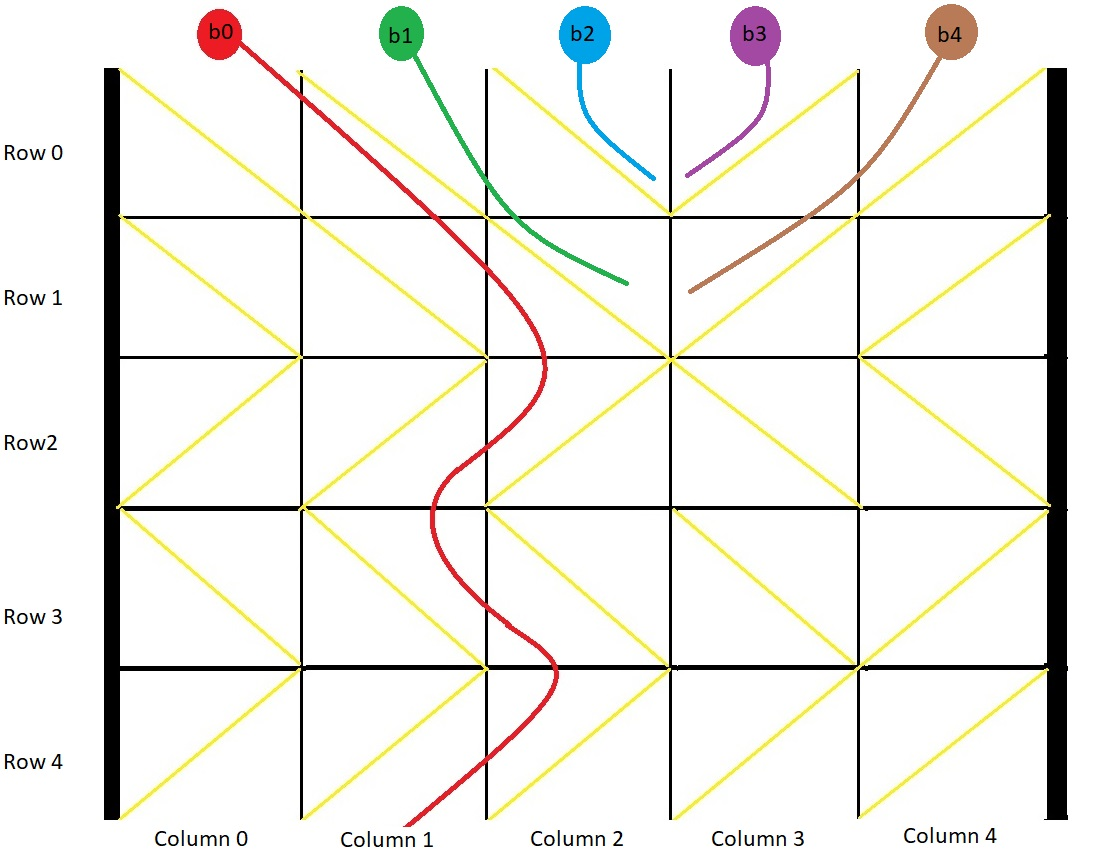1706. Where Will the Ball Fall

#### QUESTION:

You have a 2-D grid of size m x n representing a box, and you have n balls. The box is open on the top and bottom sides.

Each cell in the box has a diagonal board spanning two corners of the cell that can redirect a ball to the right or to the left.

A board that redirects the ball to the right spans the top-left corner to the bottom-right corner and is represented in the grid as 1. A board that redirects the ball to the left spans the top-right corner to the bottom-left corner and is represented in the grid as -1. We drop one ball at the top of each column of the box. Each ball can get stuck in the box or fall out of the bottom. A ball gets stuck if it hits a “V” shaped pattern between two boards or if a board redirects the ball into either wall of the box.

Return an array answer of size n where answer[i] is the column that the ball falls out of at the bottom after dropping the ball from the ith column at the top, or -1 if the ball gets stuck in the box.

Example 1:``````Input: grid = [[1,1,1,-1,-1],[1,1,1,-1,-1],[-1,-1,-1,1,1],[1,1,1,1,-1],[-1,-1,-1,-1,-1]]
Output: [1,-1,-1,-1,-1]
Explanation: This example is shown in the photo.
Ball b0 is dropped at column 0 and falls out of the box at column 1.
Ball b1 is dropped at column 1 and will get stuck in the box between column 2 and 3 and row 1.
Ball b2 is dropped at column 2 and will get stuck on the box between column 2 and 3 and row 0.
Ball b3 is dropped at column 3 and will get stuck on the box between column 2 and 3 and row 0.
Ball b4 is dropped at column 4 and will get stuck on the box between column 2 and 3 and row 1.
``````

Example 2:

``````Input: grid = [[-1]]
Output: [-1]
Explanation: The ball gets stuck against the left wall.
``````

Example 3:

``````Input: grid = [[1,1,1,1,1,1],[-1,-1,-1,-1,-1,-1],[1,1,1,1,1,1],[-1,-1,-1,-1,-1,-1]]
Output: [0,1,2,3,4,-1]
``````

Constraints:

``````m == grid.length
n == grid[i].length
1 <= m, n <= 100
grid[i][j] is 1 or -1.
``````

#### EXPLANATION:

1. 在col 0 的时候值是-1, 说明被卡住了
2. 在col n 的时候值是1, 也说明被墙壁卡住了
3. 也就是v的情况下, 也会被卡
这3种情况剔除了之后, 就可以将每一个球的情况模拟出来了. 添加到result中即可.

#### SOLUTION:

``````class Solution {
func findBall(_ grid: [[Int]]) -> [Int] {
var res = [Int]()
let rows = grid.endIndex, cols = grid.endIndex
next: for i in 0..<cols {
var currRow = 0, currCol = i
while currRow < rows {
if grid[currRow][currCol] == 1 {
guard currCol + 1 < cols else {
res.append(-1)
continue next
}
if grid[currRow][currCol+1] == 1 {
currRow += 1
currCol += 1
} else {
res.append(-1)
continue next
}
} else {
guard currCol-1 >= 0 else {
res.append(-1)
continue next
}
if grid[currRow][currCol-1] == -1 {
currRow += 1
currCol -= 1
} else {
res.append(-1)
continue next
}
}
}
res.append(currCol)
}
return res
}
}
``````